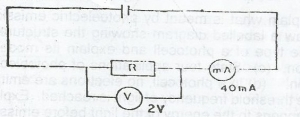# Using the data in the circuit illustrated above, calculate the value of R

Question 1Using the data in the circuit illustrated above, calculate the value of R